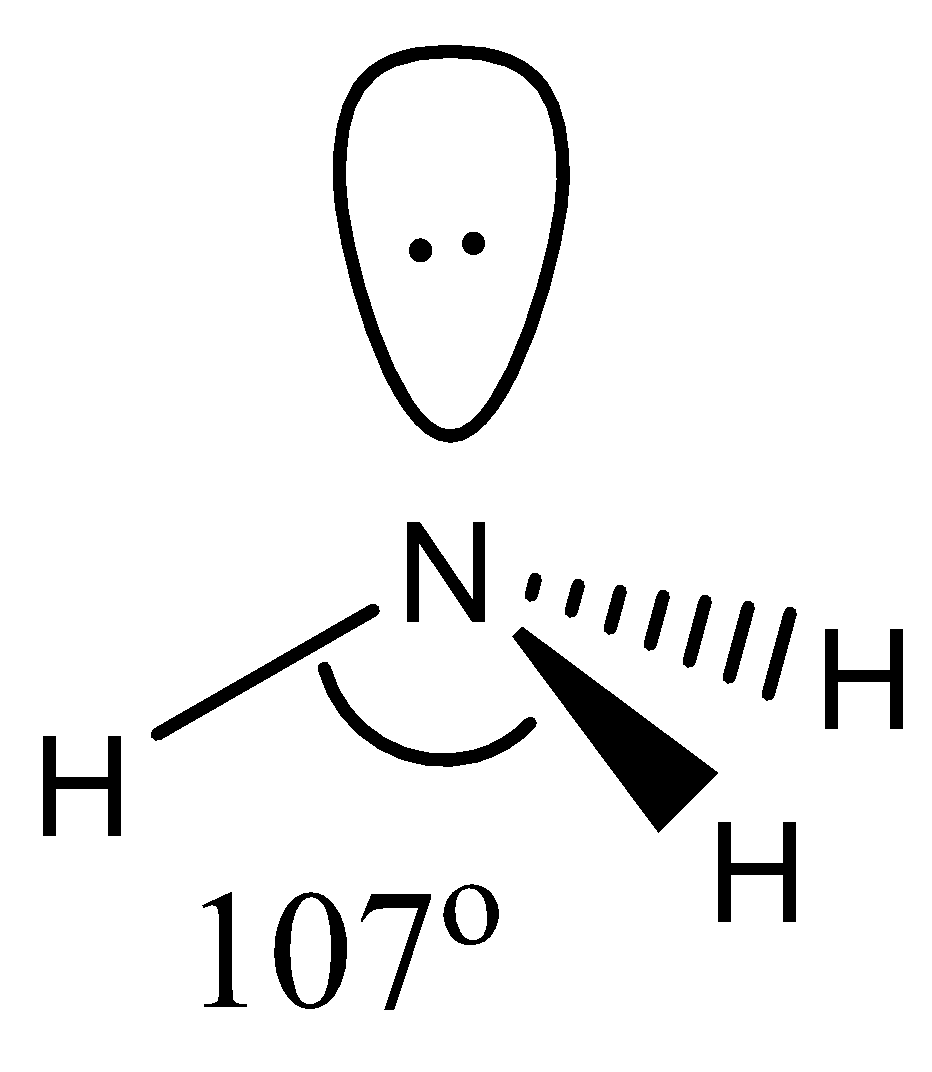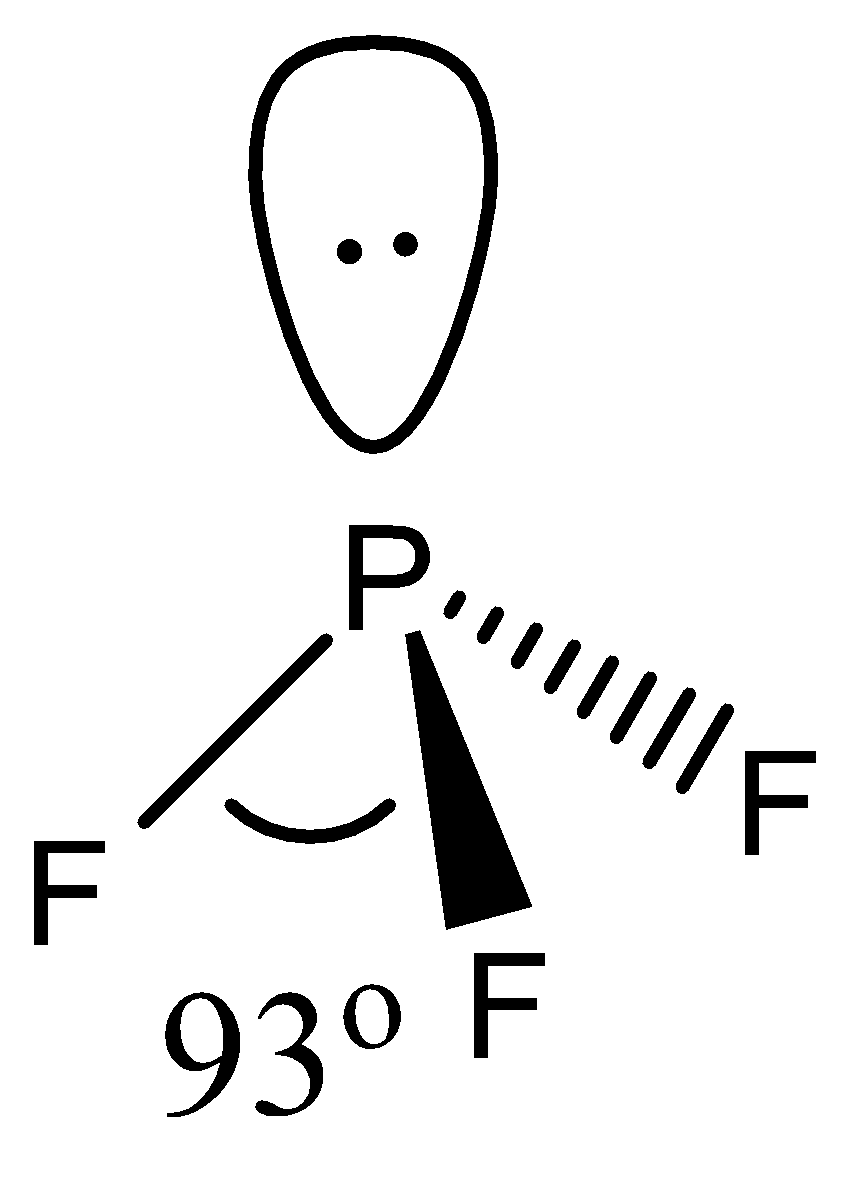QuestionAnswers

# What is the decreasing order of bond angles in $B{{F}_{3}}$, $N{{H}_{3}}$, $P{{F}_{3}}$, and ${{I}_{3}}^{-}$ ?A. $B{{F}_{3}}$ $>$ ${{I}_{3}}^{-}$ $>$ $P{{F}_{3}}$ $>$ $N{{H}_{3}}$B. ${{I}_{3}}^{-}$ $>$ $N{{H}_{3}}$ $>$ $P{{F}_{3}}$ $>$ $B{{F}_{3}}$C. $B{{F}_{3}}$ $>$ $N{{H}_{3}}$ $>$ $P{{F}_{3}}$ $>$ ${{I}_{3}}^{-}$D. ${{I}_{3}}^{-}$ $>$ $B{{F}_{3}}$ $>$ $N{{H}_{3}}$ $>$ $P{{F}_{3}}$

Hint: Think about the hybridization, 3 dimensional orientation, and number of lone pairs on each central atom to identify the basic bond angle of the structure and then move on to how the lone pairs will affect the bond angle.

First, let us look at the geometry of all the molecules:
- The geometry of $B{{F}_{3}}$ is trigonal planar. It involves 4 atoms, no lone pairs with a hybridization of $s{{p}^{2}}$. This means that the bond angle between the atoms will be $120{}^\circ$.- The geometry of $N{{H}_{3}}$ is pyramidal. Technically, the geometry is tetrahedral, but since one of the corners is occupied by a lone pair it is sometimes called pyramidal. It involves 4 atoms, 1 lone pair with a hybridization of $s{{p}^{3}}$. This means that the bond angle will be less than $109{}^\circ$ due to the presence of a lone pair. It is found to be around $107{}^\circ$.- The geometry of $P{{F}_{3}}$ is again tetrahedral. It involves 4 atoms, 1 lone pair with a hybridization of $s{{p}^{3}}$.- Since ${{I}_{3}}^{-}$ contains only 3 atoms, despite having an extra electron, its geometry is linear. Hence, the bond angle is around $180{}^\circ$.Hence, the answer is ‘D. ${{I}_{3}}^{-}$ $>$ $B{{F}_{3}}$ $>$ $N{{H}_{3}}$ $>$ $P{{F}_{3}}$’.

Note:
The bond angle of $P{{F}_{3}}$ is even smaller than $N{{H}_{3}}$. This happens due to the larger size of $F$ as compared to $H$. $F$ also repels the lone pair present on $P$ more strongly than $H$due to its own 3 lone pairs.